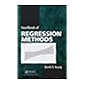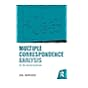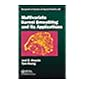Normal view MARC view ISBD view

# Extending the linear model with R: generalized linear, mixed effects and non parametric regression models

Material type:BookPublisher: New York CRC Press, Taylor and Francis 2016Edition: 2nd ed.Description: xiii, 399 p.ISBN: 9781498720960.DDC classification: 519.538 Summary: Features Offers an outstanding practical survey of statistical methods extended from the regression model Presents all of the linear model extensions using a common framework, making estimation, inference, and model building and checking clearly understandable Includes an introductory chapter that reviews the linear model and the basics of using R Provides a companion website featuring all of the datasets used in the book Summary Linear models are central to the practice of statistics and form the foundation of a vast range of statistical methodologies. Julian J. Faraway's critically acclaimed Linear Models with R examined regression and analysis of variance, demonstrated the different methods available, and showed in which situations each one applies. Following in those footsteps, Extending the Linear Model with R surveys the techniques that grow from the regression model, presenting three extensions to that framework: generalized linear models (GLMs), mixed effect models, and nonparametric regression models. The author's treatment is thoroughly modern and covers topics that include GLM diagnostics, generalized linear mixed models, trees, and even the use of neural networks in statistics. To demonstrate the interplay of theory and practice, throughout the book the author weaves the use of the R software environment to analyze the data of real examples, providing all of the R commands necessary to reproduce the analyses. All of the data described in the book is available at http://people.bath.ac.uk/jjf23/ELM/ Statisticians need to be familiar with a broad range of ideas and techniques. This book provides a well-stocked toolbox of methodologies, and with its unique presentation of these very modern statistical techniques, holds the potential to break new ground in the way graduate-level courses in this area are taught. https://www.crcpress.com/Extending-the-Linear-Model-with-R-Generalized-Linear-Mixed-Effects-and/Faraway/p/book/9781584884248
Tags from this library: No tags from this library for this title.
Item type Current location Collection Call number Status Date due Barcode
Books Vikram Sarabhai Library
General Stacks
Non-fiction 519.538 F2E9-2016 (Browse shelf) Available 193519
##### Browsing Vikram Sarabhai Library Shelves , Shelving location: General Stacks , Collection code: Non-fiction Close shelf browser519.536 Y6H2 Handbook of regression methods 519.537 H5M8 Multiple correspondence analysis for the social sciences 519.537 W4S7 Spatio-temporal statistics with R 519.538 F2E9-2016 Extending the linear model with R: generalized linear, mixed effects and non parametric regression models 519.538 H4A2 Advanced analysis of variance 519.54 C4M2 Mathematical statistics with resampling and R 519.54 C4M8 Multivariate kernel smoothing and its applications

1. INTRODUCTION

2. BINOMIAL DATA
Challenger Disaster Example
Binomial Regression Model
Inference
Tolerance Distribution
Interpreting Odds
Prospective and Retrospective Sampling
Estimation Problems
Goodness of Fit
Prediction and Effective Doses
Overdispersion
Matched Case-Control Studies
3.

COUNT REGRESSION
Poisson Regression
Rate Models
Negative Binomial
4.

CONTINGENCY TABLES
Two-by-Two Tables
Larger Two-Way Tables
Matched Pairs
Three-Way Contingency Tables
Ordinal Variables
5.

MULTINOMIAL DATA
Multinomial Logit Model
Hierarchical or Nested Responses
Ordinal Multinomial Responses
6.

GENERALIZED LINEAR MODELS
GLM Definition
Fitting a GLM
Hypothesis Tests
GLM Diagnostics

7. OTHER GLMS
Gamma GLM
Inverse Gaussian GLM
Joint Modeling of the Mean and Dispersion
Quasi-Likelihood

8.
RANDOM EFFECTS
Estimation
Inference
Predicting Random Effects
Blocks as Random Effects
Split Plots
Nested Effects
Crossed Effects
Multilevel Models

9. REPEATED MEASURES AND LONGITUDINAL DATA
Longitudinal Data
Repeated Measures
Multiple Response Multilevel Models

10.
MIXED EFFECT MODELS FOR NONNORMAL RESPONSES
Generalized Linear Mixed Models
Generalized Estimating Equations

11. NONPARAMETRIC REGRESSION
Kernel Estimators
Splines
Local Polynomials
Wavelets
Other Methods
Comparison of Methods
Multivariate Predictors

Additive Models Using the gam Package
Alternating Conditional Expectations

13. TREES
Regression Trees
Tree Pruning
Classification Trees

14. NEURAL NETWORKS
Statistical Models as NNs
Feed-Forward Neural Network with One Hidden Layer
NN Application
Conclusion
15.

APPENDICES
Likelihood Theory
R Information
Bibliography
Index

Features

Offers an outstanding practical survey of statistical methods extended from the regression model
Presents all of the linear model extensions using a common framework, making estimation, inference, and model building and checking clearly understandable
Includes an introductory chapter that reviews the linear model and the basics of using R
Provides a companion website featuring all of the datasets used in the book
Summary

Linear models are central to the practice of statistics and form the foundation of a vast range of statistical methodologies. Julian J. Faraway's critically acclaimed Linear Models with R examined regression and analysis of variance, demonstrated the different methods available, and showed in which situations each one applies.

Following in those footsteps, Extending the Linear Model with R surveys the techniques that grow from the regression model, presenting three extensions to that framework: generalized linear models (GLMs), mixed effect models, and nonparametric regression models. The author's treatment is thoroughly modern and covers topics that include GLM diagnostics, generalized linear mixed models, trees, and even the use of neural networks in statistics. To demonstrate the interplay of theory and practice, throughout the book the author weaves the use of the R software environment to analyze the data of real examples, providing all of the R commands necessary to reproduce the analyses. All of the data described in the book is available at http://people.bath.ac.uk/jjf23/ELM/

Statisticians need to be familiar with a broad range of ideas and techniques. This book provides a well-stocked toolbox of methodologies, and with its unique presentation of these very modern statistical techniques, holds the potential to break new ground in the way graduate-level courses in this area are taught.

https://www.crcpress.com/Extending-the-Linear-Model-with-R-Generalized-Linear-Mixed-Effects-and/Faraway/p/book/9781584884248

There are no comments for this item.